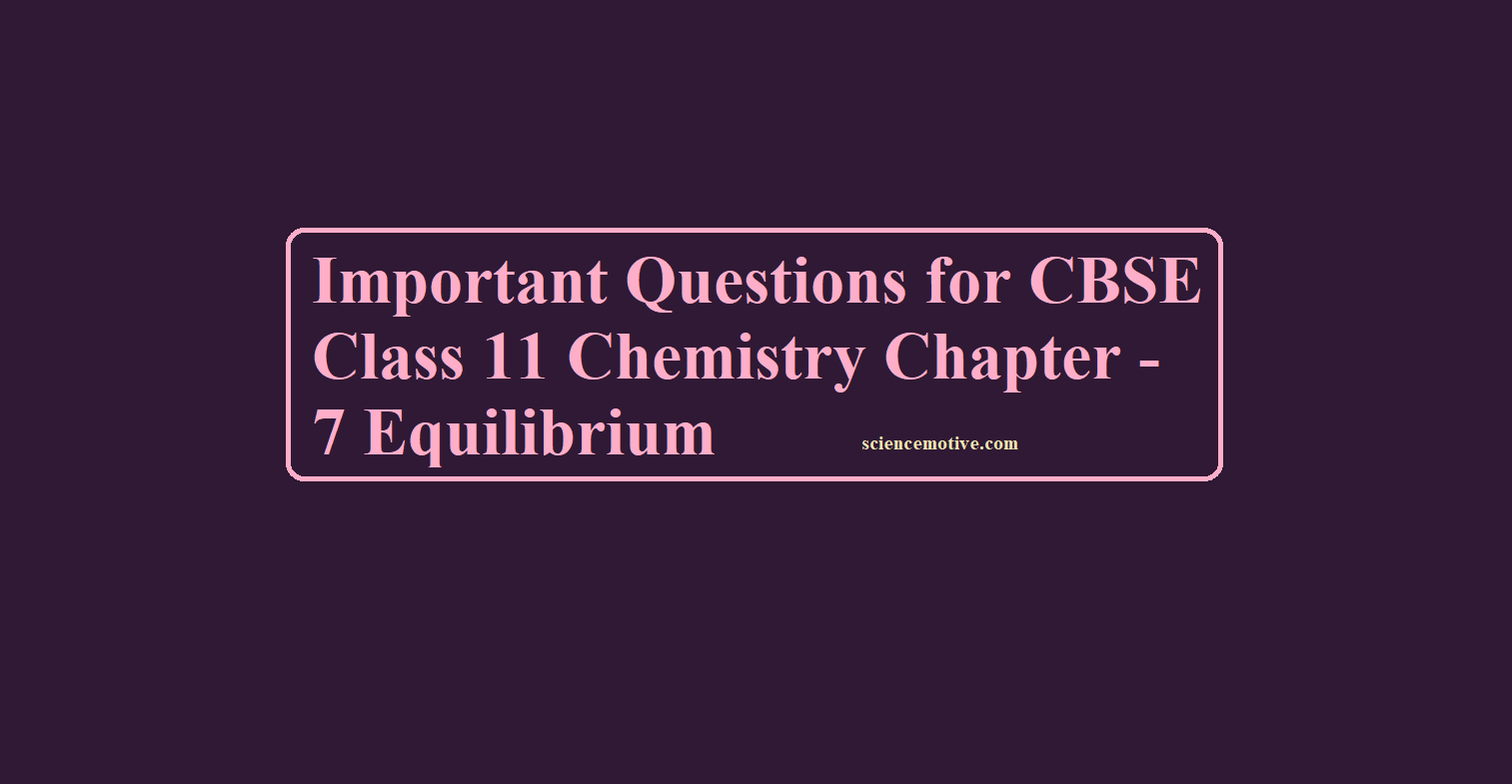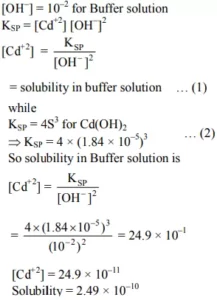# CBSE Class 11 Chemistry Chapter – 7 Important Questions# Important Questions for CBSE Class 11 Chemistry Chapter – 7 Equilibrium

Important Questions for CBSE Class 11 Chemistry Chapter – 7  Equilibrium

Que 1. For an exothermic reaction, what happens to the equilibrium constant if the temperature is raised?
Ans 1.  For an exothermic reaction, Kb will increase with rising in temperature much more than Kf. As a result, Kf/Kb will decrease.

Que 2. Ice melts slowly at higher altitudes, explain why?
Ans 2. Ice (s) → Water
The melting of ice is favored at high pressure because of the decrease in volume in the forward reactions. Since at high altitudes, atmospheric pressure is low, and therefore, ice melts slowly.

Que 3. Mention the factors that affect equilibrium constant.
Ans 3. Temperature, pressure, catalyst, and molar concentration of reactants and products.

Que 4. The molar solubility of Cd(OH)2 is 1.84 × 10-5M. Calculate the expected solubility of Cd(OH)2 in a buffer solution of pH = 12.
Ans 4. Cd(OH)2 ↔ Cd2+ + 2OHQue 5. Under what condition, a reversible process become irreversible?
Ans 5. If one of the products (gaseous) is allowed to escape out (i.e., in an open vessel) or the reaction results in the formation of the precipitate.

Que 6. At 0 0C, ice and water are present in equilibrium. What will happen on increasing the pressure?
Ans 6. On increasing the pressure, ice melts to form waver (because water has lesser volume than ice).

Que 7. What are ionic products of water?
Ans 7. Kw = [H+] [OH]

Que 8. When two reactants A and B are mixed to give product ‘c’ and ‘p’ the reaction quotient ‘Q’ at the initial stages of the reaction will be?
Ans 8. At the beginning of the reaction Q = 0. As the reaction proceeds in the forward direction, Q starts increasing. At chemical equilibrium Q = K

Que 9. What will be the pH of 1 M Na2SO4 solution?
Ans 9. Na2SO4 is a salt of strong acid and strong base and its aqueous solution will be neutral. Therefore, its pH will be 7.

Que 10. Why solution of sugar in water does not conduct electricity whereas that of common salt in water does?
Ans 10. Common salt (NaCl) is an electrolyte that in the aqueous solutions gives Na+ and Cl ions. Hence, it conducts electricity. Sugar is sucrose (C12H22O11) which is a non-electrolyte and does not give ions in the solutions. Hence, it does not conduct electricity.

Que 11. What are Buffer solutions?
Ans 11. The solutions which resist change in pH on dilution or with the addition of small amounts of acid or alkali are called Buffer Solutions.

Que 12. Calculate the pH of 0.001 M HCl solution.
Ans 12. 0.001 M HCl = 10–3 M HCl
HCl → H+ + Cl
10–3 M 10–3 M
pH = – log[H+] = – log10–3
pH = 3 (log 10 = 1)

Important Questions for CBSE Class 11 Chemistry Chapter – 7 Equilibrium

Que 13. When steam is passed over heated iron filings in an open tube, iron is completely converted into its oxide but when the tube is closed, some iron remains unreacted. Why?
Ans 13. Iron reacts with steam to form an oxide of iron (Fe3O4) and H2 gas. When the tube is open, H2 escapes and reverse reaction cannot occur but when the tube is closed, reverse reaction, i.e., the reaction between Fe3O4 and H2 also occurs forming iron and H2O vapor, and ultimately equilibrium is attained.

Que 14. State Le Chatelier’s principle.
Ans 14. It states that a change in any of the factors that determine the equilibrium conditions of a system will cause the system to change in such a manner so as to reduce or counteract the effect of the change.

Que 15. Classify the following species into Lewis acids and Lewis bases and show how these act as such: (a) HO (b)F (c) H+ (d) BCl3
Ans 15. (a) Hydroxyl ion is a Lewis base as it can donate an electron lone pair (: OH).
(b) Fluoride ion acts as a Lewis base as it can donate any one of its four-electron lone pairs.
(c) A proton is a Lewis acid as it can accept a lone pair of electrons from bases like hydroxyl ion and fluoride ion.
(d) BCl3 acts as a Lewis acid as it can accept a lone pair of electrons from species like ammonia or amine molecules.

Que 16. Explain the following:
(i) Common ion effect
(ii) solubility products
(iii) pH
Ans 16. (i) Suppression of ionization of weak electrolytes by adding a strong electrolyte having an ion common.
(ii) Product of the molar concentrations of the ions in a saturated solution, each concentration term raised to the power equal to the no. of ions produced.
(iii) Negative logarithm of hydrogen ion concentration.

Que 17. (i) Sulphuric acid is a very strong acid, yet it can also act as a base in some reactions. Explain how?
(ii) All Bronsted acids are not Lewis acids. Explain.
Ans 17. (i) Sulphuric acid (H2SO4) is weaker acid with respect to perchloric acid (HClO4). H2SO4 can rake up a proton from HClO4 to from [H3SO4]+. Hence, it acts as a base in this reaction.
(ii) Bronsted acid can donate H+ easily but they may not be able to donate electrons e.g., HCl, H2SO4. HNO3. Therefore, all Bronsted acids are not Lewis acids.

Que 18. Dihydrogen gas is obtained from natural gas by partial oxidation with steam asper following endothermic reaction: CH4 (g) + H2O (g) CO (g) + 3H2 (g)
(a) Write an expression for Kp for the above reaction.
(b) How will the values of Kp and composition of equilibrium mixture be affected by
(i) increasing the pressure
(ii) increasing the temperature
(iii) using a catalyst?
Ans 18. (a) Kp = p(CO).p(H2)3 / p(CH4).p(H2O)
(b) (i) value of Kp will not change, equilibrium will shift in the backward direction.
(ii) Value of Kp will increase and reaction will proceed in the forward direction.
(iii) No effect.

Que 19. Define dynamic equilibrium.

Ans 19: Dynamic equilibrium is that stage of the solution when the reactants are present in a closed vessel at any particular temperature reacts with each other to give rise to the products and in this process, the concentrations of the reactants go on decreasing while of products it keeps on increasing and after some time a stage occurs where we don’t find any change in the concentration of reactants or products. This stage is called the dynamic equilibrium.

Que 20. State the law of chemical equilibrium.

Ans 20: At any temperature product of the concentrations of the reaction raised to the power of their respective stoichiometric coefficient in the balanced chemical equation which is further divided by the product of concentrations of the reactants which are also raised to their individual stoichiometric coefficients has a constant value. This is known as the equilibrium law or law of chemical equilibrium.

Important Questions for CBSE Class 11 Chemistry Chapter – 7 Equilibrium